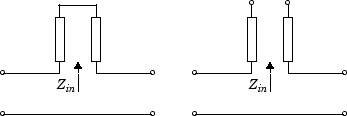# rfckt.microstrip

Microstrip transmission line

## Description

Use the `microstrip` class to represent microstrip transmission lines characterized by line dimensions and optional stub properties.

A microstrip transmission line is shown in cross-section in the following figure. Its physical characteristics include the microstrip width (w), the microstrip thickness (t), the substrate height (d), and the relative permittivity constant (ε).## Creation

### Syntax

``h = rfckt.microstrip``
``h = rfckt.microstrip(Name,Value)``

### Description

example

````h = rfckt.microstrip` returns a microstrip transmission line object whose properties are set to their default values.```
````h = rfckt.microstrip(Name,Value)` sets properties using one or more name-value pairs. For example, `rfckt.microstrip('Thickness',0.0075e-6)` creates a microstrip transmission line with thickness of `0.0075e-6` meters. You can specify multiple name-value pairs. Enclose each property name in a quote. Properties not specified retain their default values.```

## Properties

expand all

Computed S-parameters, noise figure, OIP3, and group delay values, specified as `rfdata.data` object. `AnalyzedResult` is a read-only property. For more information refer, Algorithms.

Data Types: `function_handle`

Relative permittivity of dielectric, specified as a scalar. The relative permittivity is the ratio of permittivity of the dielectric,$\epsilon$, to the permittivity in free space, ${\epsilon }_{0}$.

Data Types: `double`

Dielectric thickness or physical height of the conductor, specified as a scalar in meters.

Data Types: `double`

Physical length of transmission, specified as a scalar in meters.

Data Types: `double`

Loss angle tangent of dielectric, specified as a scalar.

Data Types: `double`

Object name, specified as an `1-by-N` character array or string scalar.

Data Types: `char`

Number of ports, specified as a positive integer. `nport`t is a read-only property.

Data Types: `double`

Conductor conductivity, specified as a scalar in Siemens per meter (S/m).

Data Types: `double`

Type of stub, specified as one of the following values: `'NotaStub'`, `'Series'`, `'Shunt'`.

Data Types: `double`

Stub transmission line termination, specified as one of the following values: `'NotApplicable'`, `'Open'`, `'Short'`.

Data Types: `double`

Physical thickness of microstrip, specified as a scalar in meters.

Data Types: `double`

Physical width of parallel-plate, specified as a scalar in meters.

Data Types: `double`

## Object Functions

 `analyze` Analyze RFCKT object in frequency domain `calculate` Calculate specified parameters for rfckt objects or rfdata objects `circle` Draw circles on Smith Chart `extract` Extract specified network parameters from rfckt object or data object `listformat` List valid formats for specified circuit object parameter `listparam` List valid parameters for specified circuit object `loglog` Plot specified circuit object parameters using log-log scale `plot` Plot circuit object parameters on X-Y plane `plotyy` Plot parameters of RF circuit or RF data on X-Y plane with two Y-axes `getop` Display operating conditions `polar` Plot specified object parameters on polar coordinates `semilogx` Plot RF circuit object parameters using log scale for x-axis `semilogy` Plot RF circuit object parameters using log scale for y-axis `smith` Plot circuit object parameters on Smith chart `write` Write RF data from circuit or data object to file `getz0` Calculate characteristic impedance of RFCKT transmission line object `read` Read RF data from file to new or existing circuit or data object `restore` Restore data to original frequencies `getop` Display operating conditions `groupdelay` Group delay of S-parameter object or RF filter object or RF Toolbox circuit object

## Examples

collapse all

Create a microstrip transmission line using `rfckt.microstrip`.

`tx1=rfckt.microstrip('Thickness',0.0075e-6)`
```tx1 = rfckt.microstrip with properties: Width: 6.0000e-04 Height: 6.3500e-04 Thickness: 7.5000e-09 EpsilonR: 9.8000 LossTangent: 0 SigmaCond: Inf LineLength: 0.0100 StubMode: 'NotAStub' Termination: 'NotApplicable' nPort: 2 AnalyzedResult: [] Name: 'Microstrip Transmission Line' ```

## Algorithms

The `analyze` method treats the microstrip line as a 2-port linear network and models the line as a transmission line with optional stubs. The `analyze` method computes the `AnalyzedResult` property of the transmission line using the data stored in the `rfckt.microstrip` object properties as follows:

• If you model the transmission line as a stubless line, the `analyze` method first calculates the ABCD-parameters at each frequency contained in the modeling frequencies vector. It then uses the `abcd2s` function to convert the ABCD-parameters to S-parameters.

The `analyze` method calculates the ABCD-parameters using the physical length of the transmission line, d, and the complex propagation constant, k, using the following equations:

`$\begin{array}{l}A=\frac{{e}^{kd}+{e}^{-kd}}{2}\\ B=\frac{{Z}_{0}*\left({e}^{kd}-{e}^{-kd}\right)}{2}\\ C=\frac{{e}^{kd}-{e}^{-kd}}{2*{Z}_{0}}\\ D=\frac{{e}^{kd}+{e}^{-kd}}{2}\end{array}$`

Z0 and k are vectors whose elements correspond to the elements of f, the vector of frequencies specified in the `analyze` input argument `freq`. Both can be expressed in terms of the specified conductor strip width, substrate height, conductor strip thickness, relative permittivity constant, conductivity, and dielectric loss tangent of the microstrip line, as described in .

• If you model the transmission line as a shunt or series stub, the `analyze` method first calculates the ABCD-parameters at the specified frequencies. It then uses the `abcd2s` function to convert the ABCD-parameters to S-parameters.

When you set the `StubMode` property to `'Shunt'`, the 2-port network consists of a stub transmission line that you can terminate with either a short circuit or an open circuit.Zin is the input impedance of the shunt circuit. The ABCD-parameters for the shunt stub are calculated as:

`$\begin{array}{c}A=1\\ B=0\\ C=1/{Z}_{in}\\ D=1\end{array}$`

When you set the `StubMode` property to `'Series'`, the 2-port network consists of a series transmission line that you can terminate with either a short circuit or an open circuit.Zin is the input impedance of the series circuit. The ABCD-parameters for the series stub are calculated as:

`$\begin{array}{c}A=1\\ B={Z}_{in}\\ C=0\\ D=1\end{array}$`

 Gupta, K. C., R. Garg, I. Bahl, and P. Bhartia, Microstrip Lines and Slotlines, 2nd Edition, Artech House, Inc., Norwood, MA, 1996.